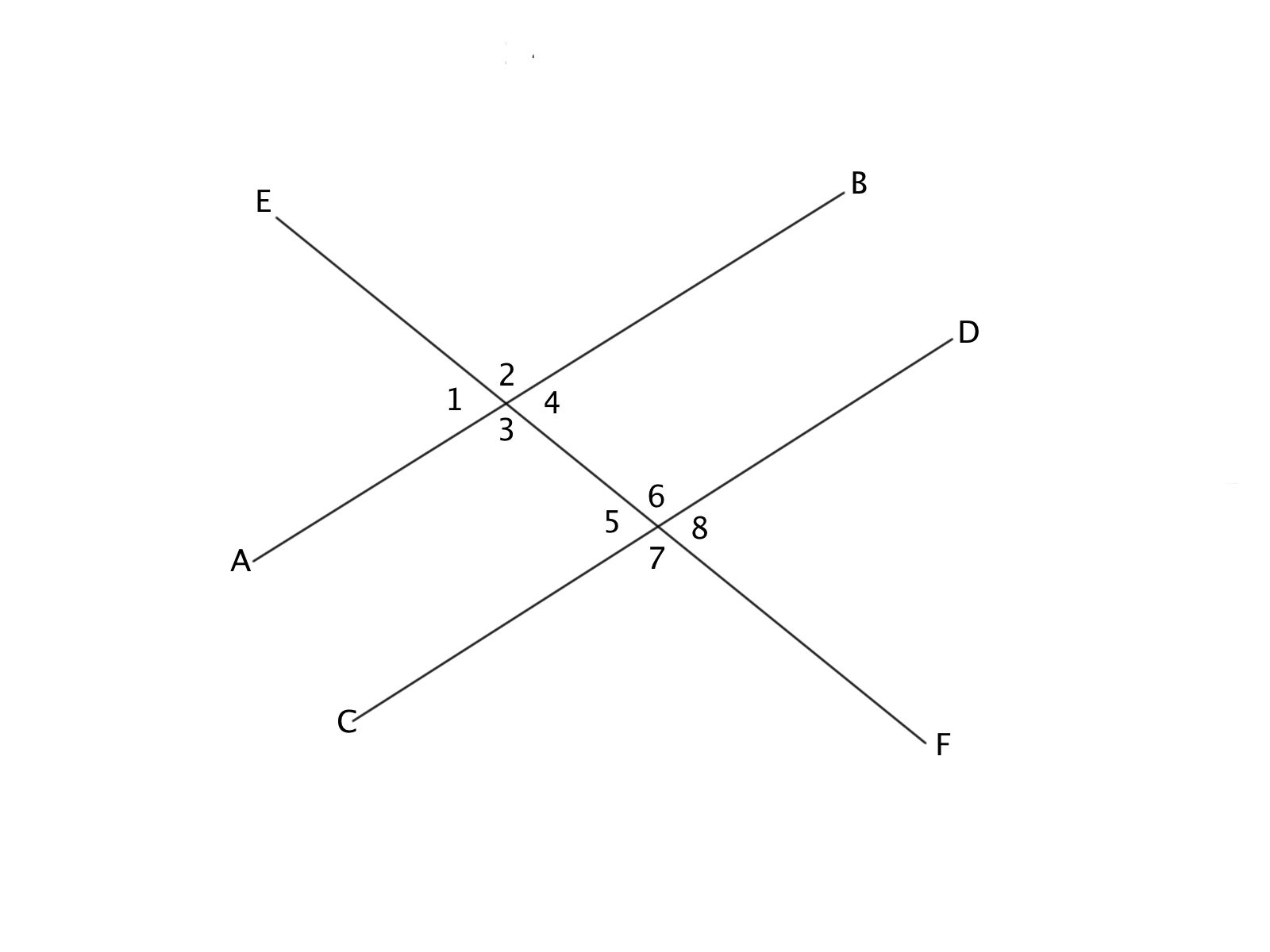### Test: Intermediate Geometry1 The slope of line CD is 4x, and Angle 1 and Angle 5 are congruent. What is the slope of line AB and why?

4x, because of Alternate Interior Angles Theorem

4x, because of Corresponding Angles Theorem

4x, because of the Vertical Angle Theorem

(1/4)x, because of the Corresponding Angle Theorem

There is not enough information to determine

1/2 questions

0%

## Access results and powerful study features!

Take 15 seconds to create an account.